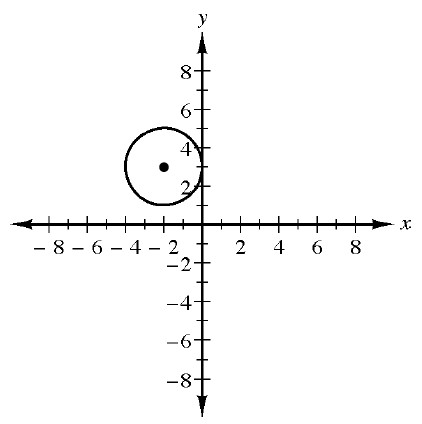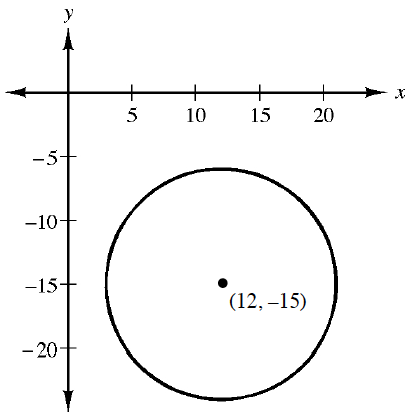### Home > CCA2 > Chapter Ch2 > Lesson 2.2.5 > Problem2-165

2-165.

Write an equation for each of the circles shown in the graphs below. 2-165 HW eTool (Desmos). Homework Help ✎.

 a.Hint (a):$(x-h)^2+(y-k)^2=r^2$More Help (a):Where is the center? How long is the radius?Answer (a):$(x+2)^2+(y-3)^2=4$ b.Hint (b):Refer to part (a).

Explore using the eTool below.
Click the link at right for the full version of the eTool: CCA2 2-165 HW eTool